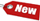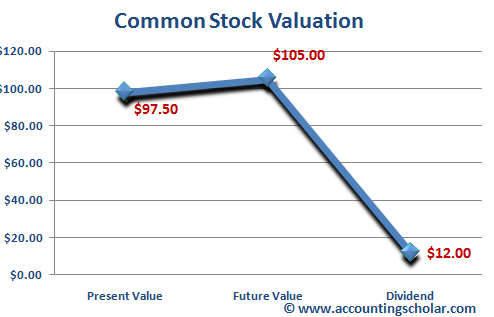Browse Accounting Lessons HereAccounting Terms & DefinitionsAccounting for Merchandising ActivitiesDebits and Credits (Double Entry Accounting)Business Valuation FormulasTime Value of Money & Present/Future ValuesComplex Debt & Equity InstrumentsCommon Stock & Shareholder's EquityAccounting & Finance RatiosValuing Common StockCorporate Income TaxesLower of Cost or Market (LCM) & Inventory ValuationChart of Accounts & BookkeepingBonds Payable & Long Term LiabilitiesCapital AssetsGAAP, Accrual & Cash Accounting, Information Commodity, Internal Controls & MaterialityWhat category of browser are you on this website? Accounting student (homework help) Finance professor (university research) Accounting manager (at work) Other Explore Careers in Accounting and FinanceVisit our section on Careers in Accounting & Finance to explore vast opportunities in this industry.

How to Value Common Stock given Required ROI (Return on Investment) and Dividends

Shares of common stock are more difficult to value than say a bond payable because of three inherent reasons:

 i) The promised cash flows from common stocks are not known in advance as opposed to bond payable where we know the cash flows. ii) The life of common stocks is forever because they have no maturity as opposed to bonds payable that have a maturity date. iii) There is no set way of coming up with a required rate of return as stocks fluctuate in value quite a bit.

Deriving the Common Stock Valuation Formula

Having said this, how can we value common stocks and discount them for the present values? Imagine that you buy a share of common stock today and plan to sell the stock in one year. From insider knowledge, you know that the stock will be worth \$80 in one year. You also think that the stock will pay \$8 per share dividend at the end of the year. If you require a 15% return on your investment, what is the most you would pay for this stock as of now? In other words, what is the present value of the \$8 dividend along with the \$80 ending value of the stock at 15% required rate of return? Here’s how to calculate this:

 Present Value = (\$8 + \$80) / 1.15 Present Value = \$76.52

Therefore, the perceived present value of this investment will be \$76.52 today. This formula can be put in more explicit terms as follows:

 P0 = (D1 + P1) / (1 + r)

Where:

 P0 = Current price of the stock P1 = Price of the stock in 1 year (or one period) D1 = Dividend expected to be paid at the end of the period (year) R = required rate of return on this investment in the market today

Example 2

From a report on a popular financial blog, you know that a stock will pay \$12 per share dividend at the end of 2009 and will be worth about \$105 on December 31st, 2009. If you require a 20% return on your investment, what is the present value of this stock that you would be willing to pay?

 P0 = (D1 + P1) / (1 + r) P0 = (\$12 + \$105) / (1 + 0.2) P0 = \$117 / 1.2 P0 = \$97.50

Therefore, we know from this that a stock that will be worth \$105 and will pay \$12 dividend at the end of the year is presently valued at \$97.50 at a discount rate of 20%.